## Milliseconds in Excel

You are reading this, so you are probably looking for some answer on how to handle milliseconds of formatting in an Excel application. All of this happens because there is neither an Excel function nor a dedicated number formatting of data in the Excel application.

I will try to show you how to create a dedicated number formatting. It will show milliseconds in your spreadsheet.

When formatting time from hours and seconds, to more advanced format like h:m:s.ms, we should do it in a step-by-step format.

## Custom number formatting

There is a way to define a custom data format in your worksheet that will allow you to display time in milliseconds. To define such a format, please follow the below steps.

Start from the empty worksheet and select an empty cell.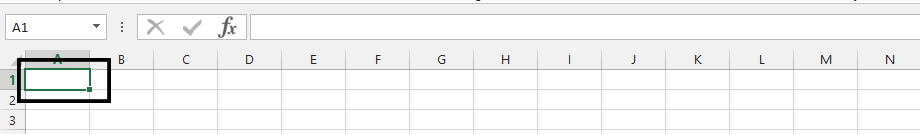Type =TIME(hour:minute:seconds)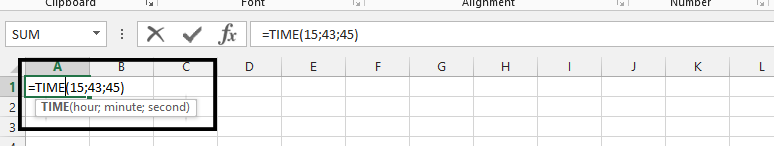Right-click on the result (1), and choose format cell (2).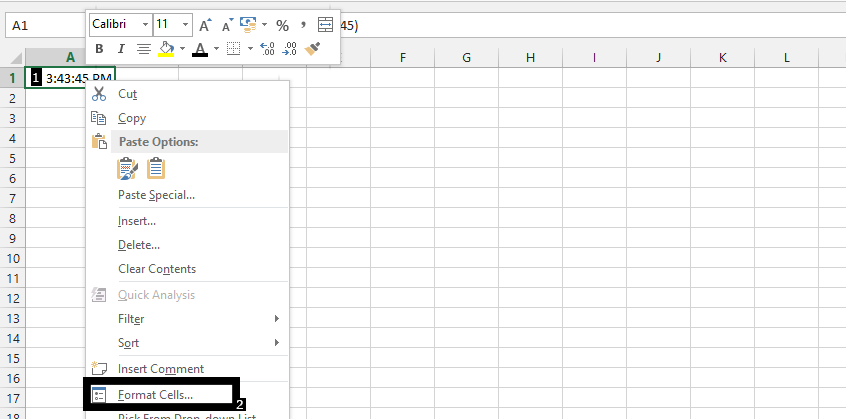Choose custom (1), write hh(tt in this case):mm:ss,000 in the field under type (2), and press ok (3).

`Note: the equivalent of such a format for VBA code would be: Cell("A1").NumberFormat = "[hh]:mm:ss.000"`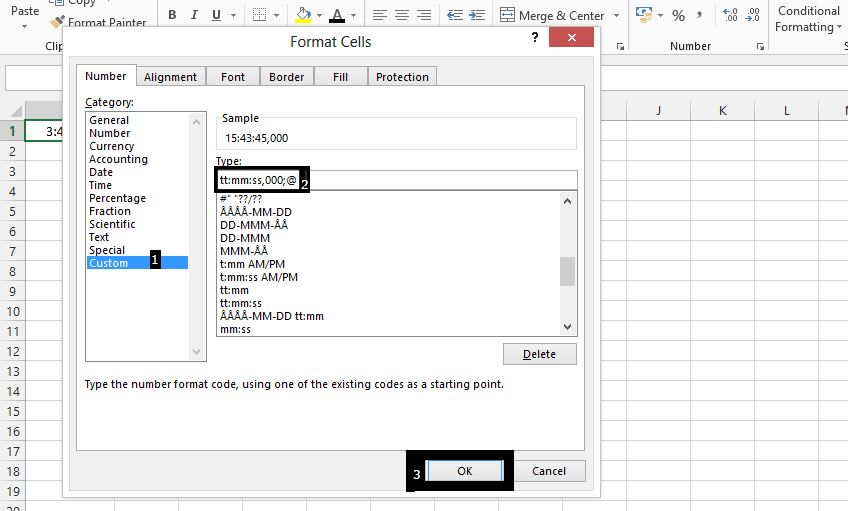The Time format would now look like this: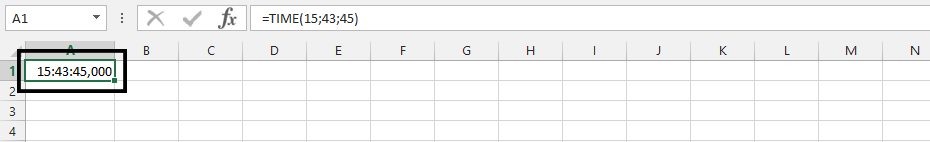Thanks to such a custom format, you are able to set accurate time and define milliseconds in your cell.

## How to format milliseconds using Vba

In Microsoft Excel, you can use Visual Basic for Applications (VBA) to format a value in milliseconds as a date and time value. Here’s an example of how to do this:

1. Open the Microsoft Excel workbook that contains the value you want to format.
2. Press Alt + F11 to open the VBA editor.
3. In the editor, insert a new module by selecting “Module” from the “Insert” menu.
4. Type the following code in the module:
``````Function FormatMilliseconds(msValue As Long) As String
FormatMilliseconds = Format(CDate("1/1/1900") + msValue / 86400000, "MM/dd/yyyy hh:mm:ss")
End Function``````
6. In a cell, enter the following formula to format a value in milliseconds as a date and time value:=FormatMilliseconds(A1)

where A1 is the cell that contains the value in milliseconds.

1. The result of the formula will be the date and time value corresponding to the number of milliseconds in the cell.

By using VBA to format values in milliseconds as date and time values, you can perform operations such as finding the difference between two dates or times, or calculating the total elapsed time between two events, and then display the results in a readable format.

## Extracting milliseconds

Let’s assume you are very precise and you need to extract only milliseconds from your data set. This task is difficult, but there is an option to display milliseconds and hide the rest.

Excel does not offer any dedicated function to manipulate milliseconds of time formatting, so we need to use usual test funtions for such purposes.

Excel formula: =RIGHT(TEXT(A2, “hh:mm:ss.000”),3) will do the trick.

Thanks to such a formula only 3 digits from the right side will be displayed and those are our milliseconds.

## How to convert a date and time value to milliseconds in Excel

In Microsoft Excel, you can represent a date and time value in milliseconds by converting it to a decimal number. The decimal portion of the number represents the time of day, and the integer portion represents the number of days since a specific starting date (usually January 1, 1900 or January 1, 1904, depending on the version of Excel being used).

Here’s an example of how to convert a date and time value to milliseconds in Excel:

1. Enter the date and time value in a cell in Excel, such as “2/13/2023 2:15:30 PM”.
2. In another cell, enter the following formula:=(A1-DATE(1900,1,1))*86400000

where A1 is the cell that contains the date and time value.

1. The result of the formula will be the number of milliseconds equivalent to the date and time value. In this example, the result would be a large number representing the number of milliseconds since January 1, 1900.

Note that this formula assumes that the date and time system in Excel is using the 1900 date system. If your Excel installation uses the 1904 date system, you would need to modify the formula accordingly.

## Things to remember:

• Custom number format for milliseconds is hh:mm:ss,000
• VBA millisecond formatting is possible thanks to Cell(“A1”).NumberFormat = “[hh]:mm:ss.000”
• To show milliseconds use =RIGHT(TEXT(A2, “hh:mm:ss.000”),3) formula

### By the way, there are:

• 60 000 milliseconds in a minute,
• 3 600 000 in hour
• 86 400 000 milliseconds in a day,
• 604 800 000 milliseconds in a week,
• 2.62974383 × 109 in a month,
• 3.1556926 × 1010 milliseconds in a year.